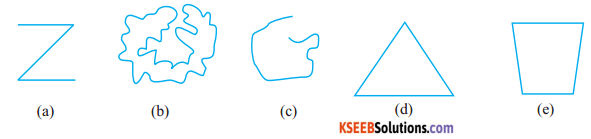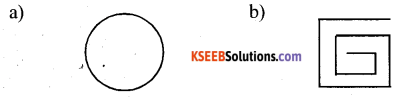KSEEB Solutions for Class 6 Maths Chapter 4 Basic Geometrical Ideas Ex 4.2

Students can Download Chapter 4 Basic Geometrical Ideas Ex 4.2 Questions and Answers, Notes Pdf, KSEEB Solutions for Class 6 Maths helps you to revise the complete Karnataka State Board Syllabus and score more marks in your examinations.

Karnataka State Syllabus Class 6 Maths Chapter 4 Basic Geometrical Ideas Ex 4.2

Question 1.
Classify the following curves as
(i) open or
(ii) Closed
Solution:Question 2.
Draw rough diagrams to illustrate the following :
a) Open curve
b) Closed curve
Solution :
a) Open curveb) Closed curveQuestion 3.
Draw any polygon and shaded its interior
Solution:Question 4.
Consider the given figure and answer the questions:
Solution:
a) Is it a curve
a) Yesb) Is it closed?
b) YesQuestion 5.
Illustrate, if possible, each one of the following with a rough diagram:
a) A closed curve that is not a polygon
b) An open curve made up entirely of line segments
c) A polygon with two sides.
Solution:c) This is not possible as the polygon having the least number of sides is a triangle Which has three side in it.

error: Content is protected !!